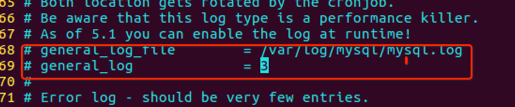# 字段查询

``````属性名称__比较运算符=值
``````

#### 查看mysql数据库日志

``````sudo vi /etc/mysql/mysql.conf.d/mysqld.conf
````````````sudo service mysql restart
``````

``````sudo tail -f /var/log/mysql/mysql.log #可以实时查看数据库的日志内容
``````

#### 条件运算符

###### 1) 查询等

exact：表示判等。

``````list=BookInfo.objects.filter(id__exact=1)

list=BookInfo.objects.filter(id=1)
``````
###### 2) 模糊查询

contains：是否包含。

``````list = BookInfo.objects.filter(btitle__contains='传')
``````

startswith、endswith：以指定值开头或结尾。

``````list = BookInfo.objects.filter(btitle__endswith='部')
``````

###### 3) 空查询

isnull：是否为null。

``````list = BookInfo.objects.filter(btitle__isnull=False)
``````
###### 4) 范围查询

in：是否包含在范围内。

``````list = BookInfo.objects.filter(id__in=[1, 3, 5])
``````
###### 5) 比较查询

gt、gte、lt、lte：大于、大于等于、小于、小于等于。

``````list = BookInfo.objects.filter(id__gt=3)
``````

``````list = BookInfo.objects.exclude(id=3)
``````
###### 6) 日期查询

year、month、day、week_day、hour、minute、second：对日期时间类型的属性进行运算。

``````list = BookInfo.objects.filter(bpub_date__year=1980)
``````

``````list = BookInfo.objects.filter(bpub_date__gt=date(1990, 1, 1))
``````

#### F对象

``````F(属性名)
``````

``````from django.db.models import F
...
``````

``````list = BookInfo.objects.filter(bread__gt=F('bcomment') * 2)
``````

#### Q对象

``````list=BookInfo.objects.filter(bread__gt=20,id__lt=3)

``````

``````Q(属性名__运算符=值)
``````

``````from django.db.models import Q
...
``````

Q对象可以使用&、|连接，&表示逻辑与，|表示逻辑或。

``````list = BookInfo.objects.filter(Q(bread__gt=20) | Q(pk__lt=3))
``````

Q对象前可以使用~操作符，表示非not。

``````list = BookInfo.objects.filter(~Q(pk=3))
``````

#### 聚合函数

``````from django.db.models import Sum
...
``````

``````  {'聚合类小写__属性名':值}
``````list = BookInfo.objects.count()